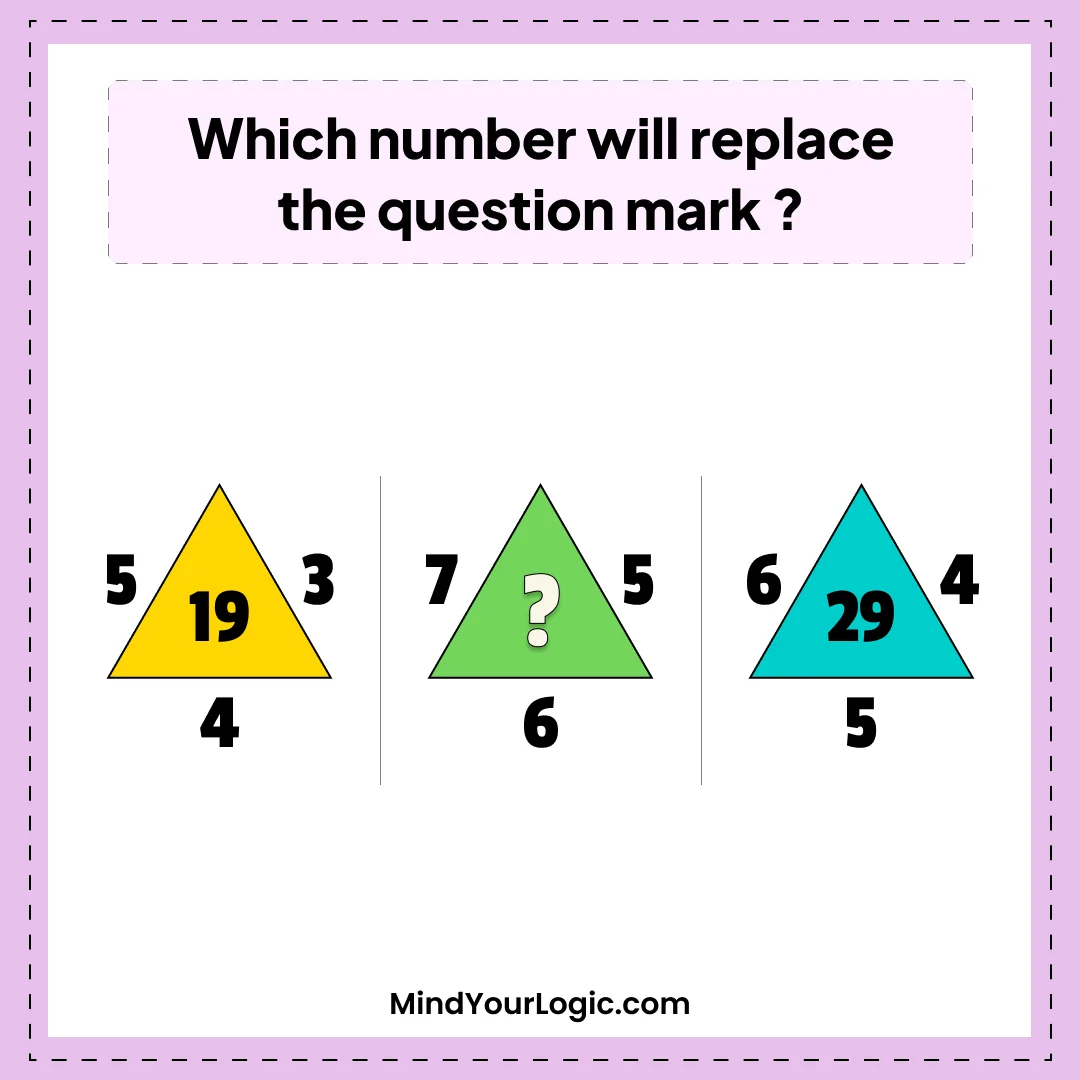# Triangle - Photo Math riddle

###### 17.Math Riddles
`Which number will replace the question mark?`• A.25
• B.37
• C.47
• D.41
• ```Explanation :

See the pattern in first triangle 5*3 +4 =19,
in the last triangle 6*4+5 = 29
then for this 7*5+6= 41```